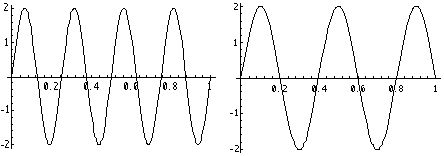# Wave Kinematics

We bring our studies to a close in this chapter about waves. They are some of the most common experiences we share: both the sounds we hear and the light we see are waves. At the same time they are subtle and pervasive: we shall see that they lie at the heart of the fundamental physics we contemplated in Chapter 7.

For our purposes, a wave is a periodic disturbance or deformation of the medium it is in. For example, sound waves are pressure variations which deform the air (or other medium) in which they travel; even empty space serves as a medium for electromagnetic waves (ie., light or radio waves). The very existence of waves is a consequence of the medium's tendency to be at rest. Once energy is transmitted to the medium, conservation of energy requires that if the medium is to come to rest at one place, it must move the energy somewhere else. That movement is the wave; we say that the wave "propagates" through the medium.

We will distinguish between several types of waves. Waves may be "transverse" or "longitudinal". Transverse waves disturb the medium at right angles to their direction of travel, while longitudinal waves disturb the medium in the same direction as they are travelling. Electromagnetic waves are transverse, while sound waves are longitudinal. We will also distinguish between travelling and "standing" waves (below). Finally, we mention that waves occur as "wavetrains", which are arbitrarily long and continuous, and as pulses, which are more compact in nature.

The propagation speed of a wave (denoted c) is the rate at which a given peak of the wave travels. The wavelength (l) is the distance between peaks, and the frequency (n = w / 2 p, where w is the "angular" frequency) is the number of peaks per unit time. The period (T = 1 / n) is the time between two peaks (recall periodic functions in math), and the wave number (k = 2p / l) is proportional to the number of peaks per unit distance. (The factors of 2p in the angular frequency and wave number are necessary so that the trigonometric functions we will be using will be periodic in the period and wavelength, respectively.) Here we see a wave train on a string as a function both of time and of distance:(The vertical scale for this example is in meters; for a sound wave, it would be in units of pressure, and for light waves it would be a field strength.) Its frequency n is 4 "Hertz" (Hz), so its period is .25 s; its wavelength is 0.4 m. Dimensional analysis gives us the "dispersion" relations

c = l n = w / k

(since c depends on the frequency, waves of different frequency tend to disperse). Therefore for this wave, c is 1.6 m / s. For sound waves in air, c is equal to the Sqrt ( 1.4 R T / (28.95 g / mol)); for electromagnetic waves, c is 1 / Sqrt (me). The relationship between the speed of light and the permittivity and permeability was one of the first clues that light is an electromagnetic wave.

Finally, the amplitude (A) of a wave is the distance from the resting (equilibrium) state of the medium to the peak of the wave. The wave above has an amplitude of 2 m. The energy of a wave is proportional to A 2, and its intensity (I) is the power it delivers per unit area (P / 4 p r 2 for a spherical wave).

It should be noted that this discussion applies to linear waves. These waves are idealizations of the waves found in nature, and are a good approximation for small amplitudes. They are called linear because they arise as solutions to linear differential equations. Other waves are "nonlinear", and their dispersion relations depend on their amplitude as well as their frequency. These waves are much more difficult to analyze, and are corresponingly more interesting! The most common examples of nonlinear waves are the "breakers" which you see on the ocean shore. We will focus on linear waves in this chapter.

There are two main types of solutions to the wave equation, as we indicated above: travelling and standing waves. Travelling waves have the form

y = A cos ( k x - w t + d).

The (dimensionless) argument of the cosine function is called the "phase", and is of the form b (x - c t); we therefore see that it describes a wave which translates to the right (for positive c) in time (recall translation of functions from algebra). Since

cos (a + b) = cos (a) cos (b) - sin (a) sin (b),

we have

y = A [cos (k x - w t) cos (d) - sin (k x - w t) sin (d)].

d is called the "phase angle", and effectively allows us to specify the relative "starting point" of the wave at time zero. By experimenting with various values of d (ie., 0, p / 2, p, 3 p / 2, 2p), we see that we can produce waves which have any given initial value (between - A and A) at time zero (see the "Travelling Waves and Beats Mathematica Notebook).

The other main type of solution to the wave equation is the standing wave, with the form

y = f (x) g (t).

As you might guess, it does not travel (translate in time), but rather oscillates "in place". We will discuss standing waves further in Section C.

The next section is about superposition.

If you have stumbled on this page, and the equations look funny (or you just want to know where you are!), see the College Physics for Students of Biology and Chemistry home page.